Free Software Development.

1. Fitting Statistical Regressions

Lorentz JÄNTSCHI

Technical University of Cluj-Napoca

Abstract

The present paper is focused on modeling of statistical data processing with applications in field of material science and engineering. A new method of data processing is presented and applied on a set of 10 Ni–Mn–Ga ferromagnetic ordered shape memory alloys that are known to exhibit phonon softening and soft mode condensation into a premartensitic phase prior to the martensitic transformation itself. The method allows to identify the correlations between data sets and to exploit them later in statistical study of alloys. An algorithm for computing data was implemented in preprocessed hypertext language (PHP), a hypertext markup language interface for them was also realized and put onto comp.east.utcluj.ro educational web server, and it is accessible via http protocol at the address http://vl.academicdirect.ro/applied_statistics/linear_regression/multiple/v1.5/.

The program running for the set of alloys allow to identify groups of alloys properties and give qualitative measure of correlations between properties. Surfaces of property dependencies are also fitted.

Keywords

Modeling, Analytical methods, Automat processing of data, Server side technologies.

1. Introduction

In field of statistical data processing it exist a large set of software to compute and fit the regressions, but few are free. Even for free software, another problem it appear, operating system license and portability of the software. As example, to use well known Microsoft Excel software, you must have Microsoft Windows and Microsoft Excel license and portability of Excel program is restricted to Windows platform. To import Excel files in another programs or platforms, conversion modules are needed, and conversion is not totally in most of the cases.

Platform independent and free software is a real alternative to this. First step to build totally free software is to install a free operating system. Unix-like operating systems are known to be free, but even here exist licensed software. In order to select a totally free Unix-like operating system, best offer come from BSD family.

The most secure and license check for installed software is NetBSD. The NetBSD detect so called “license agreement” and do not permit to install a software if the software contain unacceptable license agreement (different form free) and software can be installed only if the acceptance is explicitly stipulated by the user in configuration files of the system. Another advantage of NetBSD operating system is his huge portability under various hardware platforms from i386 family to Sun and Macintosh machines.

By another hand, most full featured operating system of BSD family is FreeBSD. One of the advantages of the FreeBSD operating system is his software portability. With adequate packages, under FreeBSD, can be executed DOS, Windows, Linux and Sun-OS programs. Another advantage of FreeBSD system comes from easiest to install and use it.

Once you have an operating system installed, the next step is to choose a proper programming language for software developing.

Here, some major questions must be answered. In terms of programming, portability of resulted program can be a problem. As example, if we are chose to implement the algorithm in Visual Basic, the execution of the program is restricted to Windows machines. If Perl is our choice, a Unix-based machine is necessary to run program.

Even if we chouse to implement the program in C language, we will have serious difficulties to compile the programs on machines running with different operating systems.

The complexity of program building is also a serious reason in language chousing. Is known that C based languages is poor in simplicity and necessity more time to expend for application building than other languages.

Other questions require an answer: We want a server based application or client based application? We want a server side application or a client side application?

As example, a client side application can have disadvantage of execution on client, and dependence of processing speed by power of client machine. If we prefer this variant, a java script or visual basic script is our programming language.

A server side application requires a web server installed. The area of web servers is also a large set, but few have multiplatform capability. If we want a full featured web server, Apache is our solution.

Under Apache, we have the possibility to execute programs already compiled in C, Fortran and Java, under Unix machines we can directly execute Perl programs, and, most important, under all operating system platforms we can execute PHP programs if we previously install php language and module binaries.

The advantage of PHP programs consist in his portability under most of operating system platforms and internal compilation feature that do not necessity the compilation “by hand” from the user. The disadvantage can be same internal compilation that consumes supplementary time in execution. But, this disadvantage can be partially eliminated through installing a PHP proxy, that store compiled programs and next execution of the unmodified program use this compiled binary. In terms of program developing PHP is easy to use, the language borrow syntax from C, Pascal, Basic and Perl, but do not borrow the complex declaration syntax from them. The pointer mechanism is absolute. Thus, a variable used as a string, can be exploited as an integer or real if the value represent a number such that. Class constructing is also available and PHP posses a strong library of database connectivity. Modular programming, recursivity and graphics are at home! Module loading of compiled programs in other languages and execution of binary programs is also available. System services such as mail service are easy to exploit in PHP scripts. A very easy mechanism to link PHP scripts to HTML scripts make PHP language to be one of the best. Shell executing commands make PHP a useful platform for system administration (PhpSysInfo, WebAdmin, PhpMyAdmin, PhpPgAdmin). As a conclusion, PHP is our choice!

PHP programs are putted on a web server data folder and executed by them using PHP module. The output of the PHP program is in HTML style and can be viewed by any web client (Microsoft Internet Explorer, Mozzilla, Opera, Netscape, Konkueror).

2. Theoretical Considerations

Many statistical procedures for processing data are available. Most of them offer a voluble set of possibilities and variants, but which one to consider them? That is not a easy question and the frequent answer is: that is choice of analyst.,

Data mining technology offer in this area of knowledge some answers, but not a complete answer. By other hand, to interpret experiment results, data need to be well processed. Structure investigations are frequently combined with statistical processing. In most of cases, best results are obtained with specific procedures in contrast to general numeric algorithms.,

Modeling of structure is benefit to property predictions., Nonstandard statistical evaluation procedures then are helpful.

The design of statistical processing program is depicted in fig. 1:Fig. 1 Program design scheme

The INPUT module read a text format data, process input data, split it into rows and columns. The rows represent the values of variables and columns represent the variables.

The INPUT module passes data in matrix form to the VARIABLES EXTRACTOR module. This module starts with pairs of columns from data matrix; continue with sets of three columns and so on, until entire set is browsed.

For every set, pass it to the SYSTEM GENERATOR. Another input in VARIABLES EXTRACTOR module comes from DECISION module. If the DECISION module detects a correlation of absolute coefficient 1 then it passes to the VARIABLES EXTRACTOR the first variable label from the regression equation and for the next extractions of variables the passed variable is marked as dependent and is not passed never again to the SYSTEM GENERATOR module.

The SYSTEM GENERATOR computes average means, needed by GAUSS module.

If name n_rows it assigned to number of rows, n_cols to number of columns, data to array of data, the output of module SYSTEM GENERATOR is computed by formulas:

M[i,j] =;

M[0,j] =, 1 ≤ i, j ≤ n_cols                                (1)

Linear regression and PLS (partial least squares) are most used methods in statistical processing of data.

Presented method (SYSTEM GENERATOR and GAUSS modules) uses them.

GAUSS module solves a linear system of equations in form: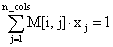, 1 ≤ i ≤ n_cols                                             (2)

If answer of algorithm solving is undetermined system and null variable is xn_cols then GAUSS module solve determined system of n_cols order given by equation (3):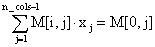, 1 ≤ i < n_cols                                  (3)

When system is solved a unique solution is found. Then, system extended matrix contain at column n_cols the coefficients of regression equation: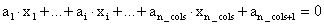(4)

where the coefficients an_cols+1 and an_cols+1 are resulted regression coefficients. Note that equation (4) is in implicit form; to obtain an explicit form is necessary to extract dependent variable from (4). The last coefficient is assigned to -1:

an­_cols+1 = -1                                                                             (5)

At the end of module SOLUTION it result an implicit linear regression equation between given variables through his values in columns (eq. 4). Equation 4 can be exploited to obtain explicit linear regression equations for each variable which has no null coefficient ai: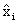=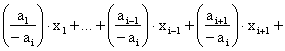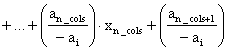(6)

Sum of residues Si can be now evaluated:

Si =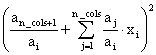(7)

To compare one equation to another, a calibration is required. Let to explicit this. If x1 values (data from input) are percents expressed in values from 0 to 100 and x2 are premartensitic temperatures transformation expressed in K with values from 100 to 600, then also sum of residues are expressed square of same measurement units.

To make independence of measurement unit and measure order, values Si are divided with own sum of squares of variable measurements (M[i,i] from INPUT module, equation 1). Final equation, with substitution xi = data[k,i],  1 ≤ k ≤ n_rows and summing is:

Qi =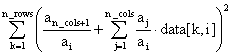/M[i,i]                     (8)

and express relative residues of variable x­i when variable xi is assumed to be dependent of independent variables x1, …, xi-1, xi+1, xcols.

Note that the dependence and independence statistical concept is hard to prove in practical situations, but will see later, can be decelerated. For a good correlation, Qi should be smallest possible value. The value of Qi is computed in SOLUTION module for every equation.

Another quantitative measure for a good correlation is correlation coefficient between measured data xi and estimated valuesfrom equation (6). Assuming that M is mean operator, for r is given by: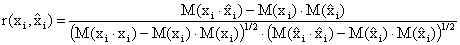(9)

The absolute value of r must be high for a good correlation. The value of r is computed in DECISION module for implicit equation and in SOLUTION module for every explicit equation. More additionally tests are also available in other programs such as Microsoft Excel or Statsoft Statistica.

3. Implementation

A graphical interface was built in PHP with a TEXTAREA for input data and an INPUT SUBMIT button for submitting data to the server. The server is a Free BSD Unix based server (5.0 DP1 software version) with an Apache web server (1.3.26 software version) running on.

The server is hosted in educational network of Technical University of Cluj-Napoca with address 193.226.7.200 and name vl.academicdirect.ro.

The PHP language was compiled with GDI (graphical device interface) and MySQL (database server) support and the PHP software version is 4.2.3.

The MySQL database server is also installed and running on and his software version is 3.23.52. The input interface is presented in fig. 2: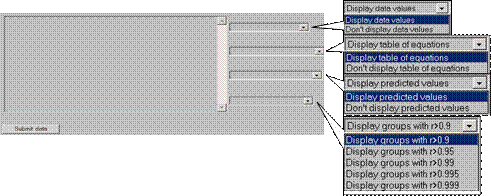Fig. 2 The output of index.php

The output of the RESULTS & GRAPHS module is passed depending on user equation selection to another PHP program that makes graphical representation of regression: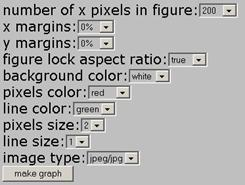Fig. 3 The output of do.php

The program build have 21 subroutines and a main program, specified below:

·      function af_ec(\$n,\$coef,&\$t) // display an equation;

·      function af_mt(\$titlu,&\$tabel,\$n_r,\$n_c) //display any matrix with a title;

·      function af_rez(\$n_r,\$n_c,&\$d,&\$m,&\$c,&\$t,\$n_o,\$pr) //list a table with best equations founded;

·      function ch_ln(\$l1,\$l2,&\$cc,\$r) // Gauss linear algebra method, change two lines in system extended matrix;

·      function cnk(\$k,\$n_r,\$n_c,&\$data,&\$tab,\$pr,&\$dep,&\$inv) //make recursive all possible combination c(n,k);

·      function data_copy(\$n_r,\$n_c,&\$d,&\$t,&\$d_t,&\$n_t) //extract a subset of data from entire set;

·      function do_means(&\$data,&\$mean,\$n_rows,\$n_cols) //make all (xi, xi*xj) means;

·      function ec_by(\$n,&\$coef,\$by,&\$c_by) //calculate coefficients for explicit equation from implicit equation coefficients;

·      function ec_val(\$n,&\$valori,&\$coef) //compute value of implicit equation in given point

·      function estimare(\$n_r,\$n_c,&\$d,&\$c,\$x,&\$x_est) //compute value for explicit equation in given point for given dependent variable;

·      function im_ln(\$nr,\$rw,&\$cc,\$r) //Gauss linear algebra method, make a unitary element into system extended matrix;

·      function mx_rw(\$cl,&\$cc,\$r) //Gauss linear algebra method, find the best line for zeroes in system extended matrix;

·      function n_to_s(\$nr) //format and display a real number;

·      function r_stat(\$n,\$k,&\$d1,&\$d2) //compute correlation coefficient r;

·      function rd_gs(\$cc,\$r,&\$cf) //Gauss linear algebra iterative algorithm;

·      function reg_lin_1(\$n_r,\$n_c,&\$d,&\$t,\$pr) //make linear regression if possible; return answer;

·      function res(&\$t,\$n) //reset counter for recursive c(n,k);

·      function sum_r(\$n_rows,\$n_cols,&\$data,&\$coef,\$cor) //calculate sum of residues;

·      function ze_pd(&\$cc,\$r) //Gauss linear algebra method, make supdiag. zeroes in system matrix;

·      function ze_sd(\$e,&\$cc,\$r) //Gauss linear algebra method, make subdiag. zeroes in system matrix;

·      main program //input data and requested minimal correlation coefficient and display founded equations;

4. Results and Discussion

The presented model make data preprocessing to a set of 10 Ni–Mn–Ga ferromagnetic ordered shape memory alloys that are known to exhibit phonon softening and soft mode condensation into a premartensitic phase prior to the martensitic transformation itself and is a extension added to the model presented in book .

The properties are described in table 2. Previous versions of this program were reported in -.

Table 1. Processed Data

 Column Property Measurement unit 1 Alloy State (Poly- or Single-crystalline alloy) 1, -1 (PC, SC respectively) 2 e/a Electron/atom ratio 3 Concentration of Ni % 4 Concentration of Mn % 5 Concentration of Ga % *6 T1 (rows 1-7), TM' (rows 8-10) K 7 TM, premartensitic temperature transformation K

*temperatures: T1= martensitic transformation; TM'=intermartensitic transformation in Group III alloys.

The input data can be copied and pasted into TEXTAREA from figure 2. By pressing submit button the input data are processed. By chousing to display data values, the input data are also displayed.

In table 2 are presented the input data, such as are showed by PHP program:

Table 2. Input data values (output by PHP program)

 0 1 2 3 4 5 6 7 1 1 7.35 49.6 21.9 28.5 4.2 178 2 1 7.36 47.6 25.7 26.7 4.2 152 3 -1 7.45 49.7 24.3 26 183 218 4 1 7.5 50.9 23.4 25.7 113 224 5 -1 7.51 49.2 26.6 24.2 184 240 6 1 7.56 47.7 30.5 21.8 227 240 7 1 7.57 51.1 24.9 24 197 248 8 -1 7.78 53.1 26.6 20.3 417 379 9 -1 7.83 51.2 31.1 17.7 443 415 10 -1 7.91 59 19.4 21.6 633 517

Program computes and output the regression equations. With an rrq = 0.9 the program found over 60 different implicit equations of linear regression with r > rrq, almost impossible to obtain by hand or in some program with statistics kernel.

If value of rrq is increased to rrq = 0.99, number of implicit equation founded is reduced at 29. For rrq = 0.999 number of implicit equation founded is 12.

That is a large set! If we are interested to study dependence between two variables form set, then we select the proper table from output of the program.

Best result is displayed in table 3, and it correlates the temperatures T1 and TM.

If we are looking for totally dependent variables (and here exists, sum of concentrations is 100%), the program finds it and also eliminate one of them from set. In table 4 is showed program response for correlating variables x2, x3 and x4 (dependent variable: x2). If we are looking for dependences between e/a and concentrations, simply select the founded equations from program output.

Table 3. Linear regression between

martensitic, premartensitic and intermartensitic temperatures

 x0 x1 x2 x3 x4 x5 x6 Equation Residue Correlation 0 0 0 0 0 1 1 +x5*1.00-x6*1.80=-2.73*102 0.37 0.98369 0 0 0 0 0 1 1 -x5*0.55+x6*1.00=+1.50*102 0.21 0.98369 0 0 0 0 0 1 1 +x5*3.66*10-3-x6*6.62*10-3=-1.00 0.42 0.98369

Table 4. Founded dependent variable

in group of concentrations of Ni(x2), Mn(x3) and Ga(x4)

 x0 x1 x2 x3 x4 x5 x6 Equation Residue Correlation 0 0 1 1 1 0 0 +x2*1.00+x3*1.00+x4*1.00=+1.00*102 0.00 1.00000 0 0 1 1 1 0 0 +x2*1.00+x3*1.00+x4*1.00=+1.00*102 0.00 1.00000 0 0 1 1 1 0 0 +x2*1.00+x3*1.00+x4*1.00=+1.00*102 0.00 1.00000 0 0 1 1 1 0 0 -x2*1.00*10-2-x3*1.00*10-2-x4*1.00*10-2=-1.00 0.00 1.00000

In table 5 is showed the dependence of e/a by concentration of Ni and Mn.

Table 5. Dependence of e/a by Ni(x2) and Mn(x3)

expressed by explicit and implicit equations

 x0 x1 x2 x3 x4 x5 x6 Equation Residue Correlation 0 1 1 1 0 0 0 +x1*1.00-x2*7.02*10-2-x3*3.99*10-2=+2.98 7.35*10-4 0.99995 0 1 1 1 0 0 0 -x1*1.42*101+x2*1.00+x3*0.56=-4.25*101 1.55*10-3 0.99997 0 1 1 1 0 0 0 -x1*2.50*101+x2*1.75+x3*1.00=-7.47*101 5.43*10-3 0.99992 0 1 1 1 0 0 0 -x1*0.33+x2*2.34*10-2+x3*1.33*10-2=-1.00 1.86*10-3 0.99995

If we are looking to express one of the temperatures depending by concentrations, then the following equations are useful (table 7):

Table 7. Dependence of TM (premartensitic temperature) by

Mn(x3) and Ga(x4) concentrations

 x0 x1 x2 x3 x4 x5 x6 Equation Residue Correlation 0 0 0 1 1 0 1 +x3*0.56+x4*1.00+x6*2.36*10-2=+4.45*101 4.88*10-2 0.99300 0 0 0 1 1 0 1 +x3*2.37*101+x4*4.23*101+x6*1.00=+1.88*103 0.16 0.99012

The equations that contain maximum of independent terms (without one concentration) is given at the end of output file of the program (table 8). Note that the variable x2 are missing from regression equation (are dependent to the x3 and x4 variables).

Table 8. Most comprehensive multi-linear dependence in data set

 x0 x1 x2 x3 x4 x5 x6 Equation Residue Correlation 1 1 0 1 1 1 1 -x0*6.77*10-4+x1*1.00+x3*2.79*10-2+x4*6.61*10-2+x5*5.27*10-6-x6*1.09*10-4=+9.82 4.12*10-4 0.99999 1 1 0 1 1 1 1 -x0*2.42*10-2+x1*3.57*101+x3*1.00+x4*2.36+x5*1.88*10-4-x6*3.92*10-3=+3.51*102 4.36*10-3 0.99995 1 1 0 1 1 1 1 -x0*1.02*10-2+x1*1.51*101+x3*0.42+x4*1.00+x5*7.97*10-5-x6*1.65*10-3=+1.48*102 1.98*10-3 0.99999 1 1 0 1 1 1 1 +x0*6.17-x1*9.11*103-x3*2.54*102-x4*6.03*102-x5*4.80*10-2+x6*1.00=-8.95*104 9.44*10-2 0.99668

In figs. 4 and 5 are plotted some selected dependences from data set. For fitting, the suggested selections from PHP program were used. Fig. 4 show a mono-variable dependence between TM and e/a, and fig. 5 show a multi-linear variable dependence between TM and alloy state (codified by -1 and 1) electron/atom ratio and composition (%Mn and %Ga).

Fig. 6 and 7 show surface dependences of T1 and respectively e/a of composition (%Ni, %Mn).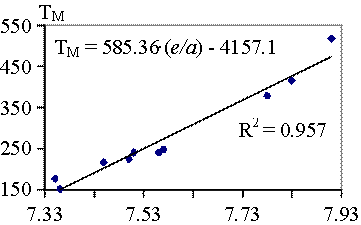Fig. 4. Regression between TM and e/a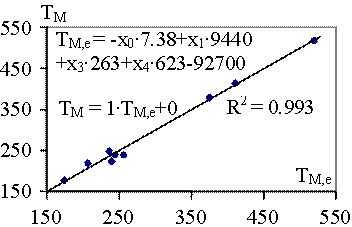Fig. 5. Regression between TM and (Alloy state, e/a, %Mn, %Ga)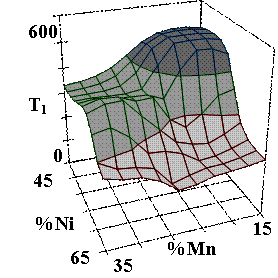Fig. 6. Surface plot of T1 and (b) e/a by composition (%Ni,%Mn)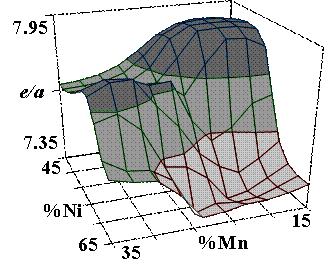Fig. 7. Surface plot of e/a by composition (%Ni,%Mn)

5. Conclusions

Considering the advantages of implemented software technology (machine and operating system portability, graphical interface and database connectivity features, easiest of programs developing, free type license agreement, http capability) the programming language and the program itself is the one of the best choice now available.

Looking to the output sums of residues from tables, is easy to observe now that the properties: type of alloy, and his martensitic, intermartensitic and premartenistic temperatures are interrelated together; these properties have the same order of sum residues in global equation, that is also expected conclusion. Very small same order of sum residues for concentrations suggest a strong interrelation between them, that is also true, because %Ni+%Mn+%Ga = 100. This conclusion lead to consider the 3D plots fitted in fig. 5 (a and b) of electron/atom ratio and T1 temperature dependencies by concentration (%Ni,%Mn). The fig. 4a prove good correlation between T1 and e/a.

6. Acknowledgments

Author is grateful to the rector of Technical University of Cluj-Napoca, prof. dr. eng. Gheorghe LAZEA for his policy on promoting information technology and also to the university staff for support related to the internet connection of comp web server.

Useful support was also benefit from Romanian Ministry of Education for finance funding of MEC/CNCSIS contract 468/"A"/2002 and 281/"A"/2002.

References

. H. Nascu, L. Jäntschi, T. Hodisan, C. Cimpoiu, G. Câmpan, Some Applications of Statistics in Analytical Chemistry, Reviews in Analytical Chemistry, Freud Publishing House, XVIII(6), 1999, 409-456.

. L. Jäntschi, M. Unguresan, MathLab. Statistical Applications, International Conference on Quality Control, Automation and Robotics, May 23-25 2002, Cluj-Napoca, Romania, work published in volume "AQTR Theta 13" (ISBN 973-9357-10-3) at p. 194-199.

. L. Jäntschi, H. Nascu, Numerical Description of Titration (MathCad application), International Conference on Quality Control, Automation and Robotics, May 23-25 2002, Cluj-Napoca, Romania, work published in volume "AQTR Theta 13" (ISBN 973-9357-10-3) at p. 259-262.

. L. Jäntschi, M. Unguresan, Parallel processing of data. C++ Applications, Oradea University Annals, Mathematics Fascicle, 2001, VIII, 105-112, ISSN 1221-1265.

. L. Marta, I. G. Deac, I. Fruth, M. Zaharescu, L. Jäntschi, Superconducting Materials: Comparision Between Coprecipitation and Solid State Phase Preparation, International Conference on Science of Materials, August 20-22, 2000, Cluj-Napoca, Romania, work published in volume 2 (ISBN 973-686-058-2) at p. 627-632.

. L. Marta, I. G. Deac, I. Fruth, M. Zaharescu, L. Jäntschi, Superconducting Materials at High Temperature: Thermal Treatment and Pb Addtition, International Conference on Science of Materials, August 20-22, 2000, Cluj-Napoca, Romania, work published in volume 2 (ISBN 973-686-058-2) at p. 633-636.

. C. Cimpoiu, L. Jäntschi, T. Hodisan, A New Method for Mobile Phase Optimization in High-Performance Thin-Layer Chromatography (HPTLC), Journal of Planar Chromatography - Modern TLC, Springer, 11(May/June), 1998, 191-194, ISSN 0933-4173.

. C. Cimpoiu, L. Jäntschi, T. Hodisan, A New Mathematical Model for the Optimization of the Mobile Phase Composition in HPTLC and the Comparision with Other Models, Journal of Liquid Chromatography and Related Technologies, Dekker, 22(10), 1999, 1429-1441, ISSN 1082-6076.

. M. Diudea, L. Jäntschi, A. Graovac, Cluj Fragmental Indices, Math/Chem/Comp'98 (The Thirteenth Dubrovnik International Course & Conference on the Interfaces among Mathematics, Chemistry and Computer Sciences), June 22-27, 1998.

. L. Jäntschi, G. Katona, M. Diudea, Modeling Molecular Properties by Cluj Indices, Commun. Math. Comput. Chem. (MATCH), 2000, 41, 151-188, ISSN 0340-6253.

. C. Sârbu, L. Jäntschi, Statistic Validation and Evaluation of Analytical Methods by Comparative Studies. I. Validation of Analytical Methods using Regression Analysis (in Romanian), Revista de Chimie, Bucuresti, 49(1), 1998, 19-24, ISSN 0034-7752.

. L. Jäntschi, Free Software Development. 3. Statistical regressions plotting with GDI interface on Apache web server and PHP module, Leonardo Electronic Journal of Practices and Technologies, Cluj-Napoca, in press.

. M. Diudea, I. Gutman, L. Jäntschi, Molecular Topology, Nova Science, Huntington, New York, 2001, 332 p., ISBN 1-56072-957-0.

. V.A. Chernenko, J. Pons, C. Seguý´, E. Cesari, Premartensitic phenomena and other phase transformations in Ni–Mn–Ga alloys studied by dynamical mechanical analysis and electron diffraction, Acta Materialia, 50, 53-60, 2002, Elsevier, USA.

. L. Jäntschi, Real Time Property Investigation in Sets of Alloys, Second International Conference on Advanced Materials and Structures, 19-21 September 2002, Timişoara, Romania, p.189-194, ISBN 973-8391-50-4.

. L. Jäntschi, Automat Server Side Processing of Statistical Data, UNITECH’02 International Conference, 21-22 November 2002, Gabrovo, Bulgaria, p. 185-189, ISBN 954-683-167-0.

. L. Jäntschi, Property Investigations with an Automat Correlation Routine and Applications for a Set of Alloys, MATEHN’02 International Conference, 12-14 September 2002, Cluj-Napoca, Romania, p. 296-301, ISSN 1221-5872.